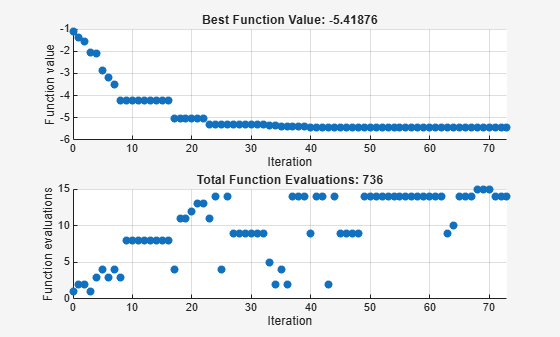# Explore `patternsearch` Algorithms in Optimize Live Editor Task

Beginning in R2022b, `patternsearch` has four algorithm options:

• `"classic"`

• `"nups"` `(Nonuniform Pattern Search)`

• `"nups-gps"`

• `"nups-mads"`

This example shows how you can try the different `patternsearch` algorithms when solving a problem using the Optimize Live Editor task.

### Specify Problem

Set up an optimization problem that has a quadratic plus linear objective function, bounds, and two linear constraints. Typically, `quadprog` is the best solver to use for this type of problem. However, this example uses `patternsearch` so you can try its different algorithms.

Set the number of variables for this problem to `N = 10`. Create a pseudorandom symmetric matrix `Q` of size `N`-by-`N` and a pseudorandom vector `z` of length `N` for the objective function `fun(x) = x*Q*x' `` N*x*z`.

```N = 10; rng default x0 = rand(1,N); x0 = x0/(2*sum(x0)); Q = 6*eye(N) + randn(N); Q = (Q + Q'); z = rand(N,1); fun = @(x)x*Q*x' - N*x*z;```

Set linear constraints on the problem: `sum(x) <= 1` and `sum(i*x) <= N/3`, where `i` is the index of the vector `x`.

```A = [ones(1,N);1:N]; % sum(x) <= 1, sum(i*x) <= N/3 b = [1;N/3];```

Confirm that `Q` is positive definite, so that the problem is convex.

`eig(Q)`
```ans = 10×1 1.5071 4.0347 7.3749 9.0561 11.6929 11.9473 13.0976 15.2099 16.1175 18.8949 ```

### Create Problem in Optimize Live Editor Task

Open a new or existing live script. In the Code section of the Live Editor tab, click Task to open the gallery of Live Editor tasks. Under Optimization, click Optimize.

#### Select Approach

In the Optimize Live Editor task, select the solver-based approach.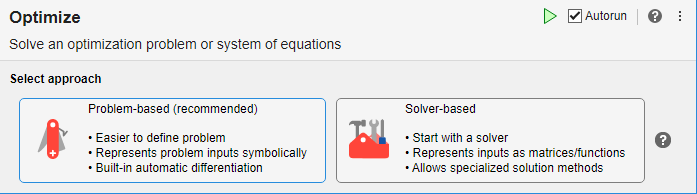#### Specify Problem Type

Select these options to specify the type of problem:

• Objective — Nonlinear

• Constraints — Lower bounds, upper bounds, and linear inequality

• Solver — patternsearch

#### Select Problem Data

Select these options for the problem data:

• Objective function — Click the From file arrow and select Function handle. Click the select arrow and select fun.

• Initial point — x0

• Constraints — Lower bounds 0

• Constraints — Upper bounds 1

• Constraints — Linear inequality constraint arrays A and b

#### Specify Solver Options

Click the arrow to expand the Specify solver options section of the task. Then, click the Add button. The task specifies the classic algorithm for the algorithm settings.

#### Display Progress

Select two plots to display: Best value and Evaluation count.

Your Optimize Live Editor task should match the one in the figure below.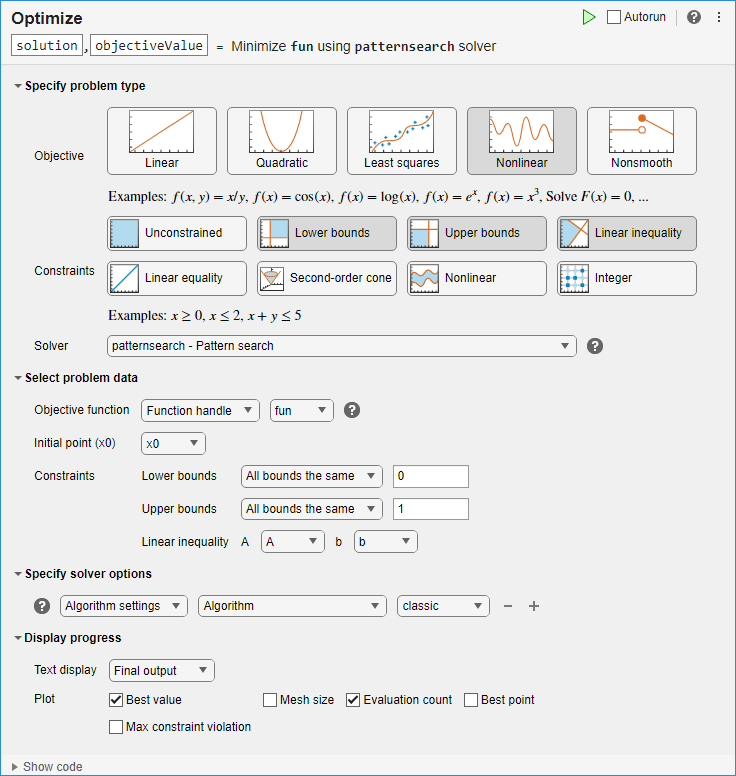### Find Solution Using Different Algorithms

After selecting the options for your problem, run the solver by clicking Run in the Run section of the Live Editor tab. The solver runs the `"classic"` `patternsearch` algorithm and displays the two specified plots.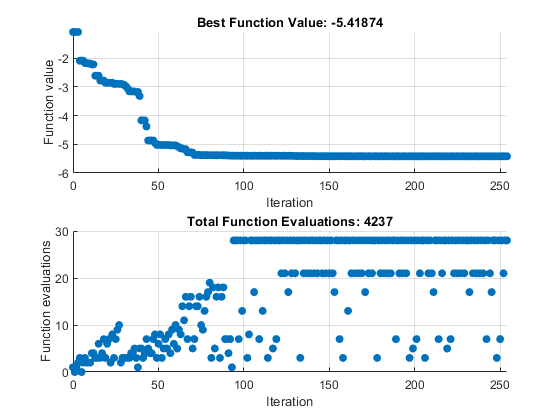Change the algorithm to nups. The solver runs the new algorithm and displays the two specified plots.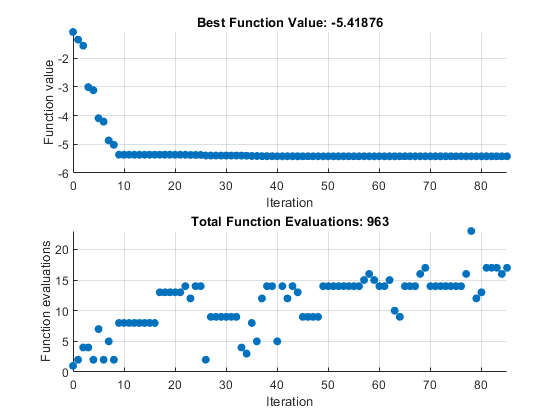The solver finishes running the `"nups"` algorithm in about a quarter of the number of function evaluations as the `"classic"` algorithm, and reaches a slightly better (lower) objective function value.

Working in the Optimize Live Editor task, you can continue to explore the other `pattersearch` algorithms and plot functions, or other options and solvers.

```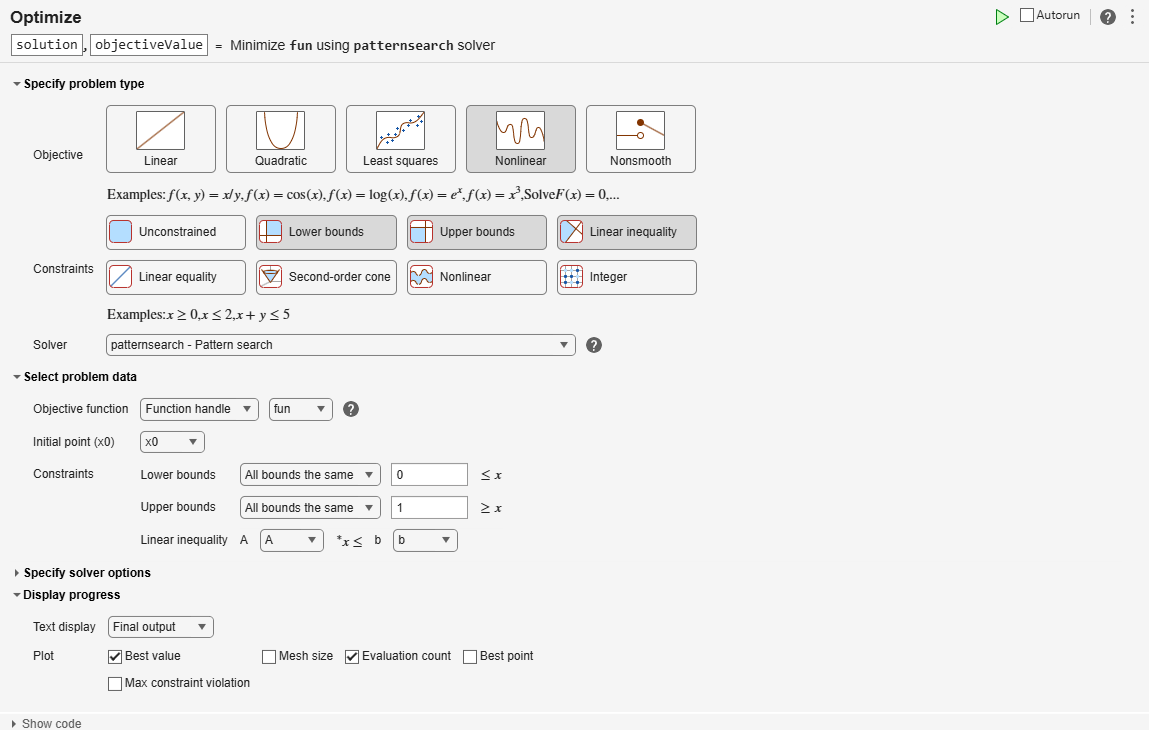```
```Optimization terminated: mesh size less than options.MeshTolerance. ```